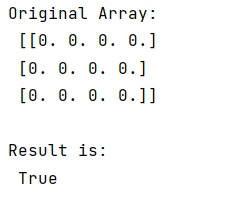# Test if NumPy array contains only zeros

Learn, how to test if NumPy array contains only zeros in Python?
Submitted by Pranit Sharma, on December 26, 2022

NumPy is an abbreviated form of Numerical Python. It is used for different types of scientific operations in python. Numpy is a vast library in python which is used for almost every kind of scientific or mathematical operation. It is itself an array which is a collection of various methods and functions for processing the arrays.

## Checking whether NumPy array contains only zeros

Suppose we need to create a NumPy array of length n, and each element of this array would be 0.

Now we need to check whether all the elements are 0 or not. The method which we will define for this purpose would return True if all the elements are 0 otherwise False.

For this purpose, we will use numpy.any() method. It is used to test whether any array element along a given axis evaluates to True and it returns a single boolean if the axis is None.

Let us understand with the help of an example,

## Python code to check whether NumPy array contains only zeros

```# Import numpy
import numpy as np

# Creating a numpy array
arr = np.zeros((3,4))

# Display original array
print("Original Array:\n",arr,"\n")

# Checking whether all elements are 0 or not
res = not np.any(arr)

# Display result
print("Result is:\n",res,"\n")
```

Output: